Unguided Volumetric Regression Net for 3D Face Reconstruction

Reconstruct a 3D facial image from a 2D facial image

Released in 2017, this net tackles the problem of regressing a 3D facial model in an end-to-end fashion, bypassing many of the difficulties found in complex pipelines involving multiple stages. Starting from a single input image, the facial model is directly reconstructed as a three-dimensional array of pixel intensities. Its architecture is based on the "hourglass" design.

Number of layers: 1,029 | Parameter count: 19,277,384 | Trained size: 81 MB

Training Set Information

• An unreleased dataset of facial images and associated 3D scans. Data was created by fitting a 3D Morphable Model (3DMM) on the 300W-LP dataset.

Performance

• This model achieves 0.0676 reconstruction accuracy on the AFLW2000-3D dataset.

Examples

Resource retrieval

Get the pre-trained net:

 In:=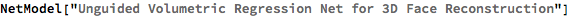Out=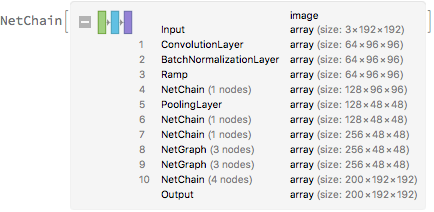Basic usage

Get the volumetric model of a facial image:

 In:=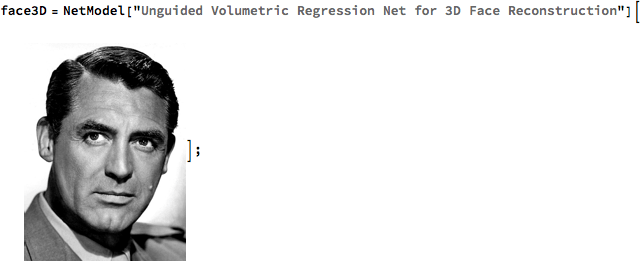Visualize the volumetric representation:

 In:=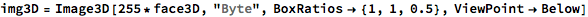Out=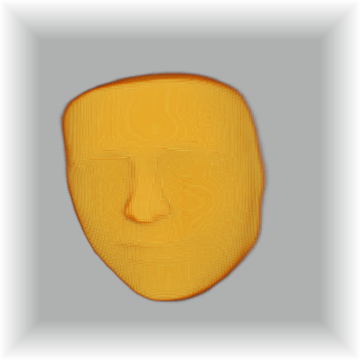Extract a 3D mesh:

 In:=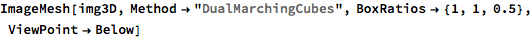Out=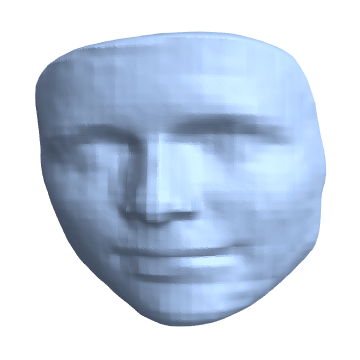Robustness to facial crop size

Get an image:

 In:=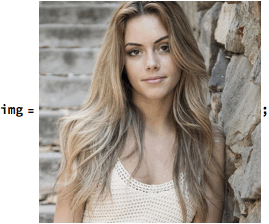Crop the image at various sizes:

 In:=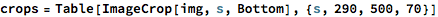Out=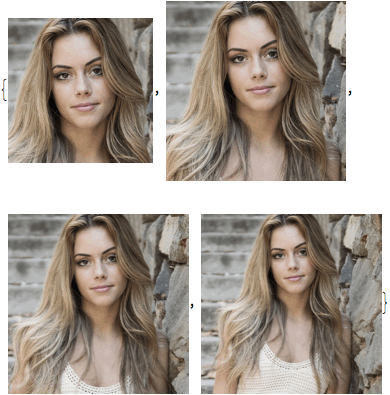Inspect the Net performance across the crops:

 In:=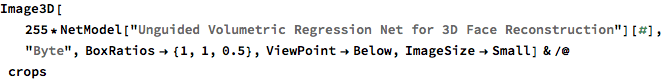Out=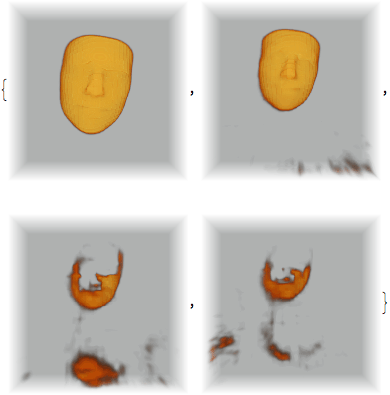Net information

Inspect the number of parameters of all arrays in the net:

 In:=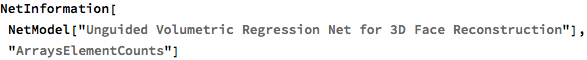Out=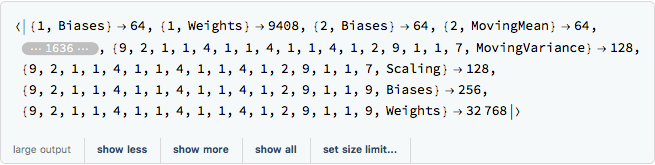Obtain the total number of parameters:

 In:=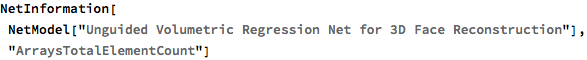Out=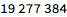Obtain the layer type counts:

 In:=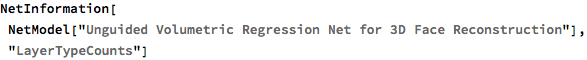Out=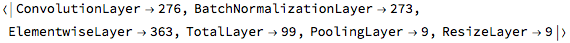Display the summary graphic:

 In:=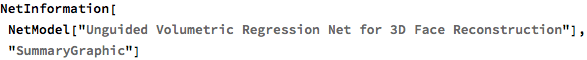Out=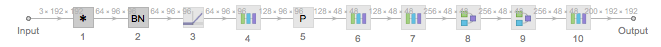Export to MXNet

Export the net into a format that can be opened in MXNet:

 In:=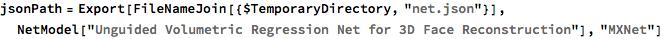Out=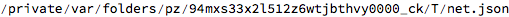Export also creates a net.params file containing parameters:

 In:=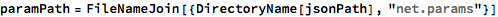Out=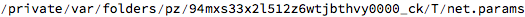Get the size of the parameter file:

 In:=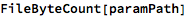Out=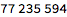The size is similar to the byte count of the resource object:

 In:=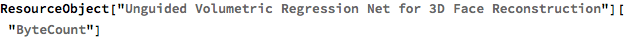Out=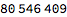Requirements

Wolfram Language 11.2 (September 2017) or above

Reference

• A. S. Jackson, A. Bulat, V. Argyriou, G. Tzimiropoulos, "Large Pose 3D Face Reconstruction from a Single Image via Direct Volumetric CNN Regression," arXiv:1703.07834 (2017)
• (available from https://github.com/AaronJackson/vrn)
• Rights: MIT License